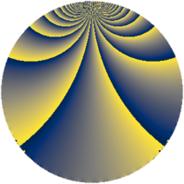# Properties

 Label 1210.2.fLevel $1210$ Weight $2$ Character orbit 1210.f Rep. character $\chi_{1210}(483,\cdot)$ Character field $\Q(\zeta_{4})$ Dimension $108$ Sturm bound $396$

# Related objects

## Defining parameters

 Level: $$N$$ $$=$$ $$1210 = 2 \cdot 5 \cdot 11^{2}$$ Weight: $$k$$ $$=$$ $$2$$ Character orbit: $$[\chi]$$ $$=$$ 1210.f (of order $$4$$ and degree $$2$$) Character conductor: $$\operatorname{cond}(\chi)$$ $$=$$ $$55$$ Character field: $$\Q(i)$$ Sturm bound: $$396$$

## Dimensions

The following table gives the dimensions of various subspaces of $$M_{2}(1210, [\chi])$$.

Total New Old
Modular forms 444 108 336
Cusp forms 348 108 240
Eisenstein series 96 0 96

## Trace form

 $$108q - 8q^{3} - 8q^{5} + O(q^{10})$$ $$108q - 8q^{3} - 8q^{5} + 8q^{12} - 108q^{16} - 8q^{20} + 16q^{23} + 32q^{25} + 8q^{26} + 16q^{27} + 16q^{31} - 124q^{36} + 32q^{37} + 24q^{38} - 40q^{42} - 56q^{45} - 24q^{47} + 8q^{48} - 56q^{53} - 8q^{56} - 32q^{58} - 24q^{60} + 24q^{67} - 32q^{70} - 96q^{71} + 72q^{75} + 16q^{78} + 8q^{80} - 188q^{81} - 16q^{82} + 64q^{86} + 32q^{91} - 16q^{92} + 56q^{93} + 24q^{97} + O(q^{100})$$

## Decomposition of $$S_{2}^{\mathrm{new}}(1210, [\chi])$$ into newform subspaces

The newforms in this space have not yet been added to the LMFDB.

## Decomposition of $$S_{2}^{\mathrm{old}}(1210, [\chi])$$ into lower level spaces

$$S_{2}^{\mathrm{old}}(1210, [\chi]) \cong$$ $$S_{2}^{\mathrm{new}}(55, [\chi])$$$$^{\oplus 4}$$$$\oplus$$$$S_{2}^{\mathrm{new}}(110, [\chi])$$$$^{\oplus 2}$$$$\oplus$$$$S_{2}^{\mathrm{new}}(605, [\chi])$$$$^{\oplus 2}$$